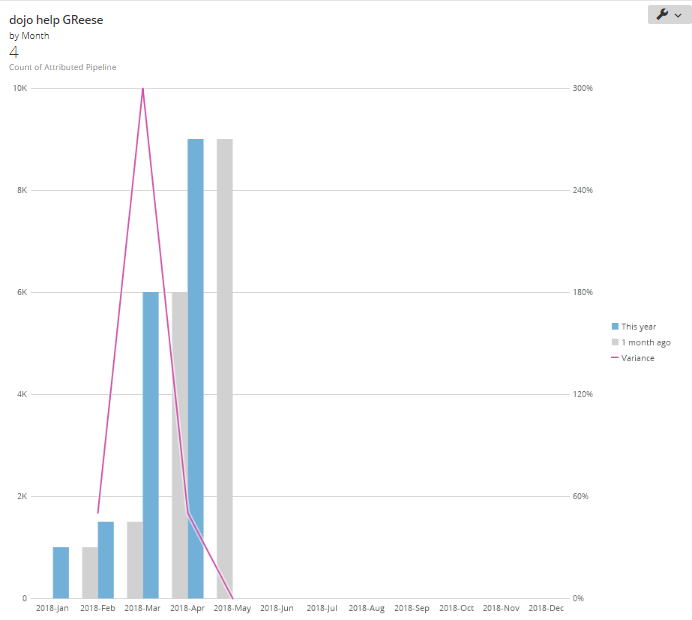# POP Beast Mode

Hoping someone can help me figure out why my POP Beast Mode calculation returns 0% in my charts. I want to compare current quarter to last quarter.  I may also modify the calculation to compare last quarter to 2 quarters ago. I am only using two fields from my dataset, similiar to this table and my dates are always the 1st day of each month.

 Month Attributed Pipeline 1/1/2018 \$1,000 2/1/2018 \$1,500 3/1/2018 \$6,000 4/1/2018 \$9,000

This is my Beast Mode calculation that returns 0% in my charts.  I tried SUM before my case statement, but that did not change the results.

(CASE  WHEN ((year(`Month`)=year(curdate())) AND (quarter(`Month`)=quarter(CURDATE()))) THEN `Attributed Pipeline` END / CASE  WHEN (quarter(curdate())=1) THEN (CASE  WHEN ((year(`Month`)=(year(curdate()) - 1)) AND (quarter(`Month`)=4)) THEN `Attributed Pipeline` END ) ELSE (CASE  WHEN ((year(`Month`)=year(curdate())) AND (quarter(`Month`)=(quarter(CURDATE()) -1))) THEN `Attributed Pipeline` END ) END) -1

Thanks in advance for any help!!

•CoachAt a high level your beast mode looks conceptually ok.  What kind of chart are you using and where is the value showing up as 0?

Is that what your data actually looks like, or do you have more rows per month that would need to be summed up?

What I'd do is break it up by the components (numerator and denominator) and then see if those values come up as expected.  Is your numerator (the sum of current quarter dollars) also zero?  Get that number right, then move on to the denominator (previous month)

`sum(CASE  WHEN year(`Month`)=year(curdate()) AND quarter(`Month`)=quarter(CURDATE()) THEN `Attributed Pipeline` END) / sum(CASE WHEN quarter(curdate())=1THEN (CASE        WHEN year(`Month`)=year(curdate()) - 1 AND quarter(`Month`)=4             THEN `Attributed Pipeline`       END)ELSE (CASE        WHEN year(`Month`)=year(curdate()) AND quarter(`Month`)=quarter(CURDATE()) -1       THEN `Attributed Pipeline`       END) END)-1`
Aaron
MajorDomo @ Merit Medical

**Say "Thanks" by clicking the heart in the post that helped you.
**Please mark the post that solves your problem by clicking on "Accept as Solution"
•CoachYou could also display the POP veriance using the Period over Period Variance bar line card:This way you wouldn't need any beast modes.  This card was made from the table you posted

“There is a superhero in all of us, we just need the courage to put on the cape.” -Superman